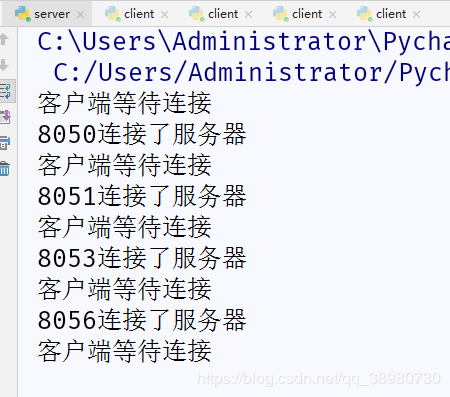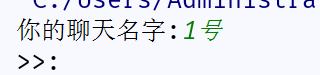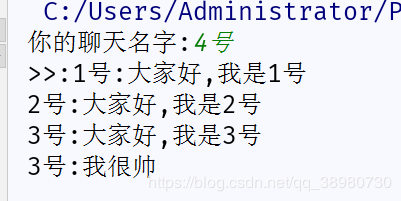# python实现简单聊天室功能 可以私聊

更新时间：2019年07月12日 14:45:20   作者：日天达人我要评论

```import socket,struct,json
def send_dic(c,dic):
dic_json=json.dumps(dic)
dic_json_length=len(dic_json.encode('utf-8'))
struct_dic_json_length=struct.pack('q',dic_json_length)
c.send(struct_dic_json_length)
c.send(dic_json.encode('utf-8'))
def get_dic(c):
try:
dic_length=struct.unpack('q',c.recv(8))
except:
return {'msg':'exit'}
try:
dic_json=c.recv(dic_length).decode('utf-8')
except:
return {'msg':'exit'}
return dic```

```import socket
import lib.common #导入写在lib里面的公共模块,代码在上面
import re
#进行开启服务器等一系列操作
s=socket.socket()
ip_host=('127.0.0.1',8000)
s.bind(ip_host)
s.listen()
#创建一个列表,用来保存客户端及其信息
c_list=[]
while True:
tag=False
dic=lib.common.get_dic(c)
if dic['msg']=='exit':
#如果接受出异常,或是客户端主动输入为exit,在列表中移除客户端信息
for i in c_list:
c_list.remove(i)
break
if dic['is_siliao']==True:
#客户端发来的字典里面如果is_siliao==True,进入私聊代码
for i in c_list:
#遍历列表,并用正则表达式截取信息
li=re.findall('(.*?)@%s(.*)'%i['name'],dic['msg'])
if len(li)!=0:
dic['msg']=li+li
lib.common.send_dic(i['client'],dic)
tag=True
break
if tag:
continue
#如果不是私聊,进入下面代码,在聊天室进行群聊
for i in c_list:
lib.common.send_dic(i['client'],dic)
while True:
#用线程池,进行多次连接
print('客户端等待连接')
name=c.recv(1024).decode('utf-8')#进行第一次接受,接受客户端的名字,为私聊的功能做准备
c_list.append(c_dic)#将字典加入列表

```import lib.common
c=socket.socket()
ip_host=('127.0.0.1',8000)
c.connect(ip_host)
def send_msg(c,name):
while True:
msg = input ('>>:').strip ()
is_siliao=False
if not msg:
continue
# if msg.startswith('@'):
if '@'in msg:
is_siliao=True
dic = {'msg': msg,'name':name,'is_siliao':is_siliao}
lib.common.send_dic(c,dic)
if msg=='exit':
c.close ()
break
def get_msg(c):
while True:
dic=lib.common.get_dic(c)
if dic['is_siliao']==True:
print('来自%s的私聊:%s'%(dic['name'],dic['msg']))
continue
print('%s:%s'%(dic['name'],dic['msg']))
name=input('你的聊天名字:').strip()
c.send(name.encode('utf-8'))
t.submit(send_msg,c,name)
t.submit(get_msg,c)```